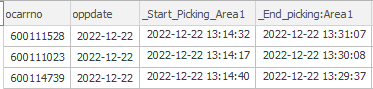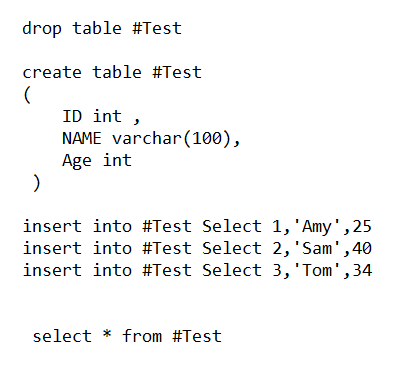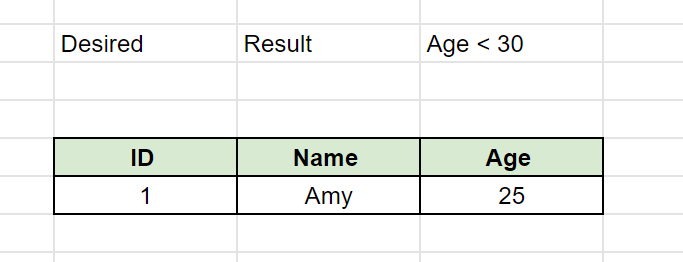# Two selects in same query

Hello!
I have an query that shows the following

1. What time did picking start and finished for an order in a specific area, in this case (fmha) Area1

I would like to add the same select for the same order one more time in the same query, but then from another area, for example Area 2

How is this best achieved? I have tried union, but didnt succed to get it correct

This is how it looks nowBut I want it to be like this

this is the current query

``````SELECT ocarrno,
oppdate,
Min(datreg)                             '_Start_Picking_Area1',
Max(datreg)                             '_End_picking:Area1',
Cast(Max(datreg) - Min(datreg) AS TIME) '_Tidsskillnad',
fmha
FROM   astro_view_cnt_svl16001
WHERE  datreg < Dateadd(d, Datediff(d, 0, Getdate()), 1)
AND datreg > Dateadd(d, Datediff(d, 0, Getdate()), -1)
AND l16lcode = 4
AND fmha = 'AREA1'
GROUP  BY oppdate,
ocarrno,
fmha
ORDER  BY Max(datreg) DESC
``````

Looking at this .. makes no sense at all

Please provide sample data and DDL

I only have one table where I get the data from (ASTRO_VIEW_CNT_SVL16001)

So from this table I see that first pick in AREA1 in fmha was first done 05:14:06, and last pick 06:07:40. But this table could also contain picking on the same order but in an diferent area, for example AREA2 in fmha. So if there have been done picking in another area to, then I want that area to be displayed to with timestamps. The result im looking for

``````Sample data
ocarrno    	oppdate    	datreg    			fmha    		l16lcode
406360150	2022-12-22	07:15:00			AREA2  			4
406360150	2022-12-22	07:00:00			AREA2			4
406360150	2022-12-22	06:07:40			AREA1  			4
406360150	2022-12-22	05:14:06			AREA1  			4

``````

hi

hope this helps

assumption = only 2 records for AREA1 and 2 records for AREA2
for the same ocarno , oppdate

This is one way of doing this

There are other ways also .. I mean the SQL that is written

create sample data

drop table #SampleData
create table #SampleData(ocarrno int, oppdate date, datereg time , fmha varchar(10), l16lcode int)
insert into #SampleData select 406360150, '2022-12-22','07:15:00','AREA2',4
insert into #SampleData select 406360150, '2022-12-22','07:00:00','AREA2',4
insert into #SampleData select 406360150, '2022-12-22','06:07:40','AREA1',4
insert into #SampleData select 406360150, '2022-12-22','05:14:06','AREA1',4
select * from #SampleData

``````; with cte as
(
select
ROW_NUMBER() over ( order by   ocarrno ,  oppdate, fmha) as rn
,  ocarrno
,  oppdate
,  min(datereg) as min_datereg
,  max(datereg) as max_datereg
,  fmha
from
#SampleData
group by
ocarrno
,  oppdate
, fmha
)
select
a.ocarrno
, a.oppdate
, a.min_datereg as Start_Picking_Area1
, a.max_datereg as End_Picking_Area1
, b.min_datereg as Start_Picking_Area2
, b.max_datereg as End_Picking_Area2
from
cte a
join
cte b
on a.rn+1 = b.rn
``````

Thanks! But it doesnt really seems to work as I wanted it to work.

For example

1. Order 600128157 according to Data, it was first picked in Area "PFZ1" 06:49 and last picked 06:52 in that area.

2. Then picked in Area PFZ2 06:59

The only correct value the sql shows is for the area PFZ2

I guess that there needs to be defined that for examample "Area2" = only orders picked in fmha PFZ1

fmha= the area

Not sure I understand

Please provide Sample Data with DDL and provide Result Set you wantSo there is one table that contains when and where the order was picked. This example have been picked in 4 different areas

hi

i gave it a shot

hope this is the correct solution

create sample data

drop table #SampleData

create table #SampleData(ocarrno int, oppdate date, datereg time , fmha varchar(10), l16lcode int)

insert into #SampleData select 406360150, '2022-12-22','10:35:00','BBY',4
insert into #SampleData select 406360150, '2022-12-22','10:30:00','BBY',4

insert into #SampleData select 406360150, '2022-12-22','10:25:00','PCH',4
insert into #SampleData select 406360150, '2022-12-22','10:20:00','PCH',4

insert into #SampleData select 406360150, '2022-12-22','10:15:00','HF',4
insert into #SampleData select 406360150, '2022-12-22','10:10:00','HF',4

insert into #SampleData select 406360150, '2022-12-22','10:05:00','LF',4
insert into #SampleData select 406360150, '2022-12-22','10:00:00','LF',4

``````; WITH cte AS
(
SELECT
ocarrno
,  oppdate
,  l16lcode
,  fmha
,  min(datereg) as [Start]
,  max(datereg) as [End]
FROM
#SampleData
GROUP BY
ocarrno
,  oppdate
,  l16lcode
,  fmha
)
SELECT
ocarrno
,  oppdate
,  l16lcode
, max(case when fmha = 'BBY' then  Start  end)   as Start_BBY
, max(case when fmha = 'BBY' then  [End]  end)   as End_BBY
, max(case when fmha = 'PCH' then  Start  end)   as Start_PCH
, max(case when fmha = 'PCH' then  [End]  end)   as End_PCH
, max(case when fmha = 'HF'  then  Start  end)   as Start_HF
, max(case when fmha = 'HF'  then  [End]  end)   as End_HF
, max(case when fmha = 'LF'  then  Start  end)   as Start_LF
, max(case when fmha = 'LF'  then  [End]  end)   as End_LF
FROM
cte
GROUP BY
ocarrno
,  oppdate
,  l16lcode
``````

Big thanks harishgg1!
It worked well, how do I adjust so the start and end time only shows without the date?

``````; WITH cte AS
(
SELECT
ocarrno
,  oppdate
,  l16lcode
,  fmha
,  min(datreg) as [Start]
,  max(datreg) as [End]

FROM
ASTRO_VIEW_CNT_SVL16001

AND l16lcode = 4

GROUP BY
ocarrno
,  oppdate
,  l16lcode
,  fmha
)
SELECT
ocarrno
,  oppdate
,  l16lcode
, max(case when fmha = 'PHF1' then  Start  end)   as _Starttid_PHF1
, max(case when fmha = 'PHF1' then  [End]  end)   as _Sluttid_PHF1
, max(case when fmha = 'PLF1' then  Start  end)   as _Starttid_PLF1
, max(case when fmha = 'PLF1' then  [End]  end)   as _Sluttid_PLF1
, max(case when fmha = 'PCH1'  then  Start  end)   as _Starttid_PCH1
, max(case when fmha = 'PCH1'  then  [End]  end)   as _Sluttid_PCH1

FROM
cte
GROUP BY
ocarrno
,  oppdate
,  l16lcode
``````

cast ( start ) as time

cast ( [End] as time )

Tried to add that, but can't figure out exactly where to put it in the code

``````; WITH cte AS
(
SELECT
ocarrno
,  oppdate
,  l16lcode
,  fmha
,  cast(min(datereg) as time )  as [Start]
,  cast(max(datereg) as time )  as [End]
FROM
#SampleData
GROUP BY
ocarrno
,  oppdate
,  l16lcode
,  fmha
)
SELECT
ocarrno
,  oppdate
,  l16lcode
, max(case when fmha = 'BBY' then  Start  end)   as Start_BBY
, max(case when fmha = 'BBY' then  [End]  end)   as End_BBY
, max(case when fmha = 'PCH' then  Start  end)   as Start_PCH
, max(case when fmha = 'PCH' then  [End]  end)   as End_PCH
, max(case when fmha = 'HF'  then  Start  end)   as Start_HF
, max(case when fmha = 'HF'  then  [End]  end)   as End_HF
, max(case when fmha = 'LF'  then  Start  end)   as Start_LF
, max(case when fmha = 'LF'  then  [End]  end)   as End_LF
FROM
cte
GROUP BY
ocarrno
,  oppdate
,  l16lcode
``````

Thanks!